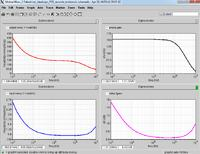# Spectre F and NF calculation wrong?

Status
Not open for further replies.

#### mtwiegI've been simulating a mixer+LNA using PNOISE in spectre, and I can't make sense of how F and NF are being calculated from the simulated input referred noise. Below is an example result, input noise and NF are at the bottom:At 1MHz, my input referred noise is Vn=0.937nV/√Hz, and my source is a 50Ω port (Vs=0.91nV/√Hz). But spectre calculates NF as 6.42dB and F as 4.39. But F should be F=1+(Vn/Vs)², where Vn and Vs are in V/√Hz, which should give F=2.06 and NF=3.14dB. From looking at different points on the plots, it seems like spectre is using the formula F=4*(Vn/Vs)². Why is this? Is it assuming a matched load on my source? My input is a relatively high impedance, so this is not the case...

Last edited:

Status
Not open for further replies.